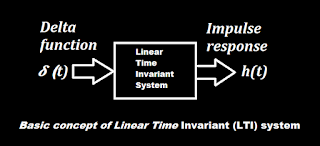# Linear Time Invariant (LTI) System

Linear Time Invariant (LTI) System

Linear Time Invariant System•Linear system means a system which possesses the property of superposition.

•Time invariant system means its behavior and characteristics are fixed over time.

•Before knowing Linear Time Invariant (LTI) system we should know what is convolution, Laplace transform and Fourier transform.

•Convolution is the mathematical way combining two signals to form third signal.

•Laplace transform is use to find transfer function by taking Laplace transform of output upon Laplace transform of input.

•Fourier transform is having the similar relation like Laplace transform.

•We can get the transfer function from the impulse response.

•Impulse response is related to frequency response via Fourier transform.

← Prev Question Next Question →